• 1）Degree_Distribution.m -- 复杂网络度分布计算的Matlab程序，这个Matlab程序是一个对复杂网络的度分布进行计算的程序，用于复杂网络的分析。 2）复杂网络节点度及度分布的MATLAB代码.txt -- 复杂网络节点度和度...
• matlab程序用于计算复杂网络度相关系数和平均聚类系数的求解。适合于编程新手使用。大家可以使用，用于社交网络中复杂网络的计算。
• 用来计算复杂网络的节点分布以及的累积概率分布（记得把矩阵内容换成自己的哦）
• 相关、不相关网络的理解（不一定正确） 社交网络中，你刚注册，系统给你推荐了小明，你关注小明的概率和小明一点关系都没有，纯粹是你为了完成任务随便选了个人关注。因为你关注小明的概率随机，只是由你自己的...

【笔记】

度相关、不相关网络的理解（不一定正确）

社交网络中，你刚注册，系统给你推荐了小明，你关注小明的概率和小明一点关系都没有，纯粹是你为了完成任务随便选了个人关注。因为你关注小明的概率随机，只是由你自己的关注总数（节点出度）决定，和对方的关注数和被关注数无关。这个网络就是度不相关网络。

你已经玩社交网络很久，系统根据你的兴趣给你推荐小明，你关注小明的概率和小明是不是大V有很大关系（假设大V发文内容有吸引力）。因为你关注大V的概率由你的关注总数，和大V的粉丝数共同决定。这个网络就是度相关网络。

同理，换成无向网络，就没有出度相关性和入度相关性的区分，统一称为度相关性。

同配、异配网络

研究表明，真实世界网络中节点之间的连接选择并不是均等的，而是存在明显的偏好．连接偏好导致网络中节点与节点之间的连接存在某种相关性，Newman根据复杂网络节点之间这种连接相关性，提出同配(assortativity)和异配(disassortativity)概念来区分节点之间的这种连接偏好，并利用匹配系数来量化节点之间的连接。

所以，复杂网络节点的异质性，是对节点分布均匀情况的一种度量。节点倾向于连接有相似度的节点，则构成同配网络；高度节点和低度节点也有一定概率连接，则构成异配网络。网络异配程度测量。

度的0阶、1阶、2阶分布特性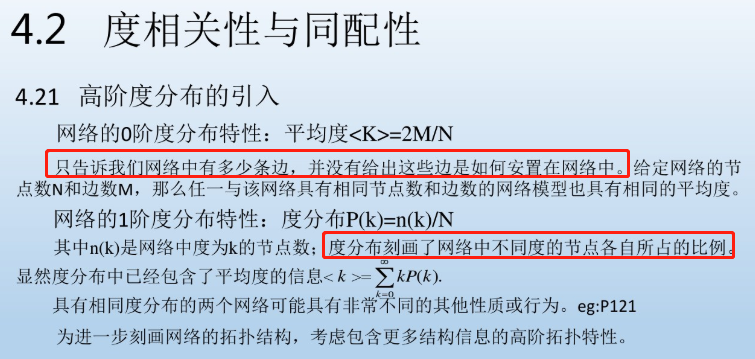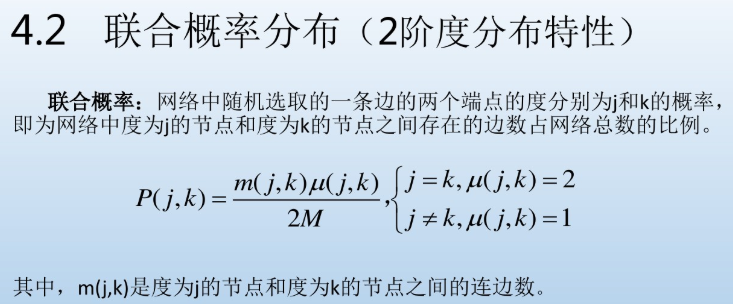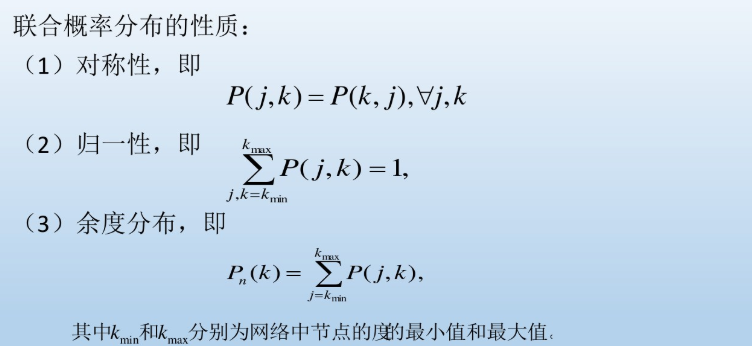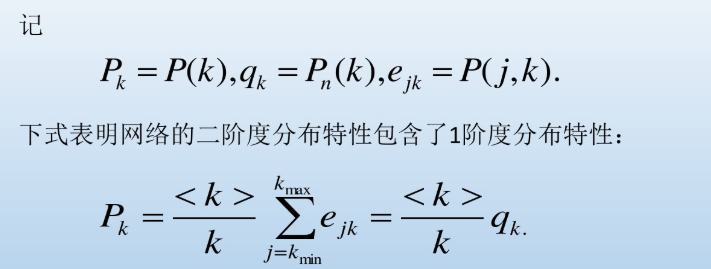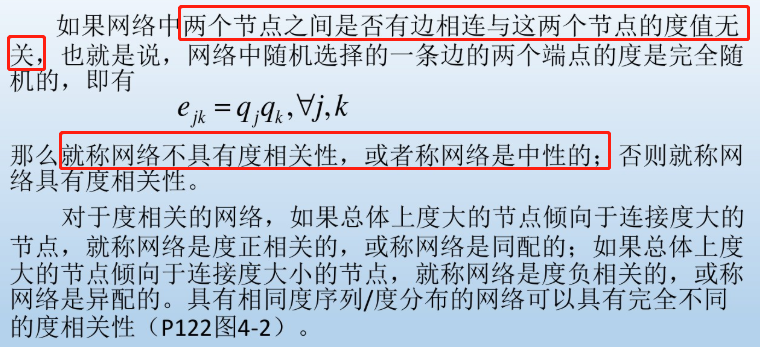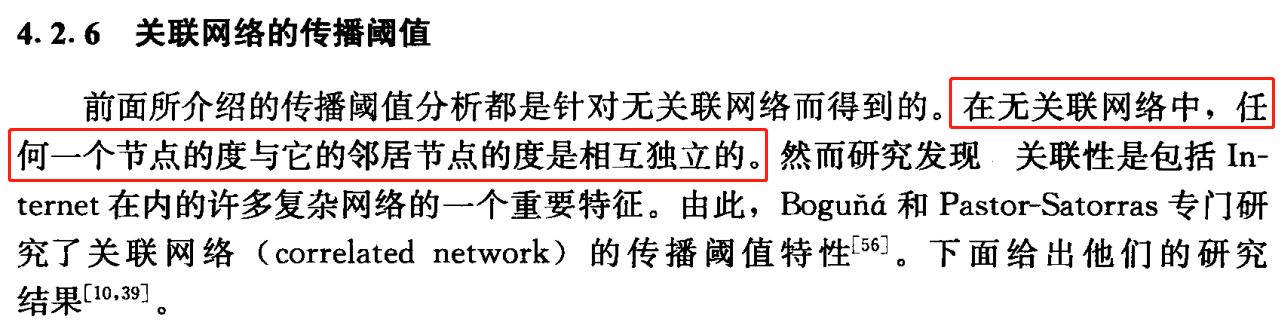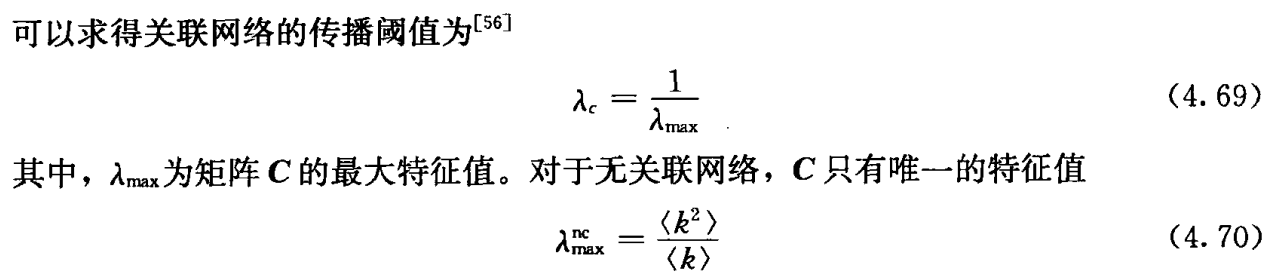来源1：复杂网络基础理论（正确）来源2：复杂系统与复杂理论（错误）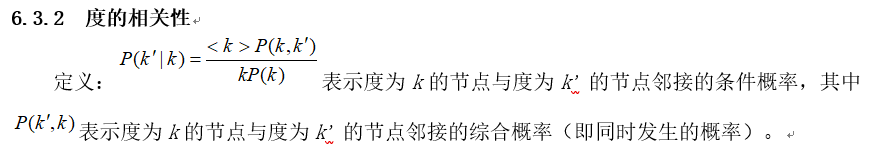展开全文• 复杂网络度分布（幂律分布）图Python 1. 无向图 直接使用networkx所提供的相关函数。 代码 import matplotlib.pyplot as plt # 导入科学绘图包 import networkx as nx import numpy as np adj = np.loadtxt('adj...

1. 无向图

直接使用networkx所提供的相关函数。

• 代码
import matplotlib.pyplot as plt  # 导入科学绘图包
import networkx as nx
import numpy as np

print("某个节点的度:", G.degree(0))  # 返回某个节点的度

# print("所有节点的度:",G.degree())#返回所有节点的度
# print("所有节点的度分布序列:",nx.degree_histogram(G))#返回图中所有节点的度分布序列（从1至最大度的出现频次）
degree = nx.degree_histogram(G)  # 返回图中所有节点的度分布序列

print(degree)
x = range(len(degree))  # 生成X轴序列，从1到最大度
y = [z / float(sum(degree)) for z in degree]  # 将频次转化为频率
plt.figure(figsize=(5.8, 5.2), dpi=150)
plt.rcParams['font.sans-serif'] = ['SimHei']  # 用来正常显示中文标签
plt.xlabel("Degree", size=14)  # Degree
plt.ylabel("Frequency", size=14)  # Frequency
plt.xticks(fontproperties='Times New Roman', size=13)
plt.yticks(fontproperties='Times New Roman', size=13)
plt.loglog(x, y, '.')
plt.show()  # 显示图表

• 效果图（与有向图 3 一致）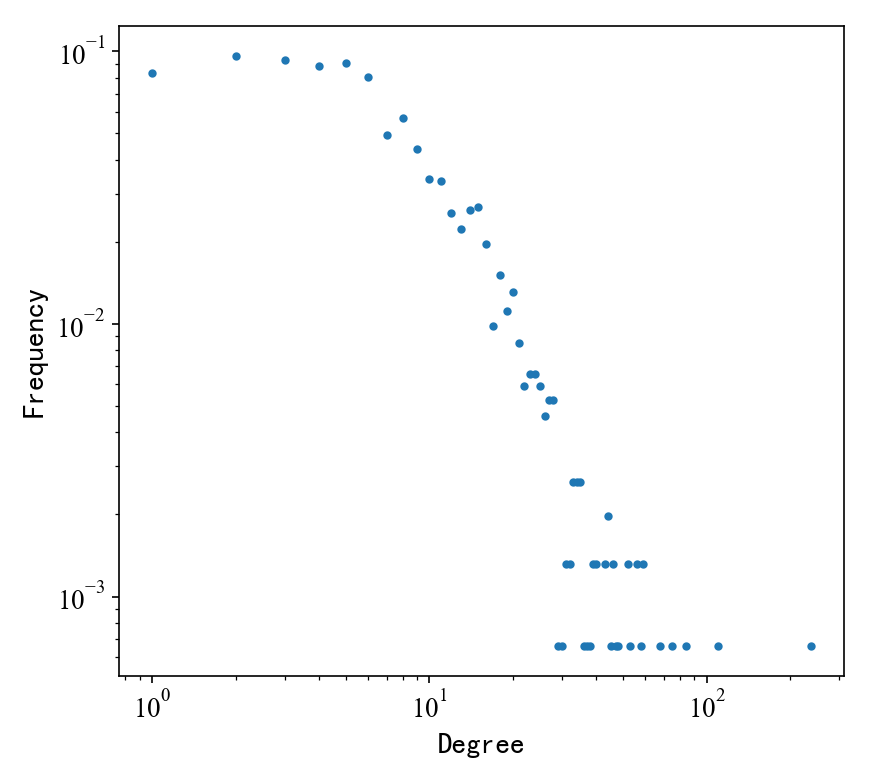2. 有向图

• 代码
import matplotlib.pyplot as plt
import numpy as np
import pandas as pd
from collections import Counter

'''
统计表方法
csv文件 每行[id,indegree,outdegree,degree]
画度分布图
'''
namelist = ['入度', '出度', '度']

for i in range(1,4):
# 数据列，
degree = np.array(data.iloc[:,[0,i]])
# 分布统计
counts = Counter(d for n, d in degree)
b = [counts.get(i, 0) for i in range(max(counts) + 1)]
x = range(len(b)) # x轴
y = [z for z in b] # y轴
# 画图
plt.figure(figsize=(5.8, 5.2), dpi=150)
plt.xlabel("Degree")
plt.ylabel("Frequency")
plt.xticks(fontproperties='Times New Roman', size=14)
plt.yticks(fontproperties='Times New Roman', size=14)
plt.loglog(x, y, '.')
plt.savefig('figure/'+namelist[i-1]+'分布图.png') # 保存图片
plt.show()

• 效果图
入度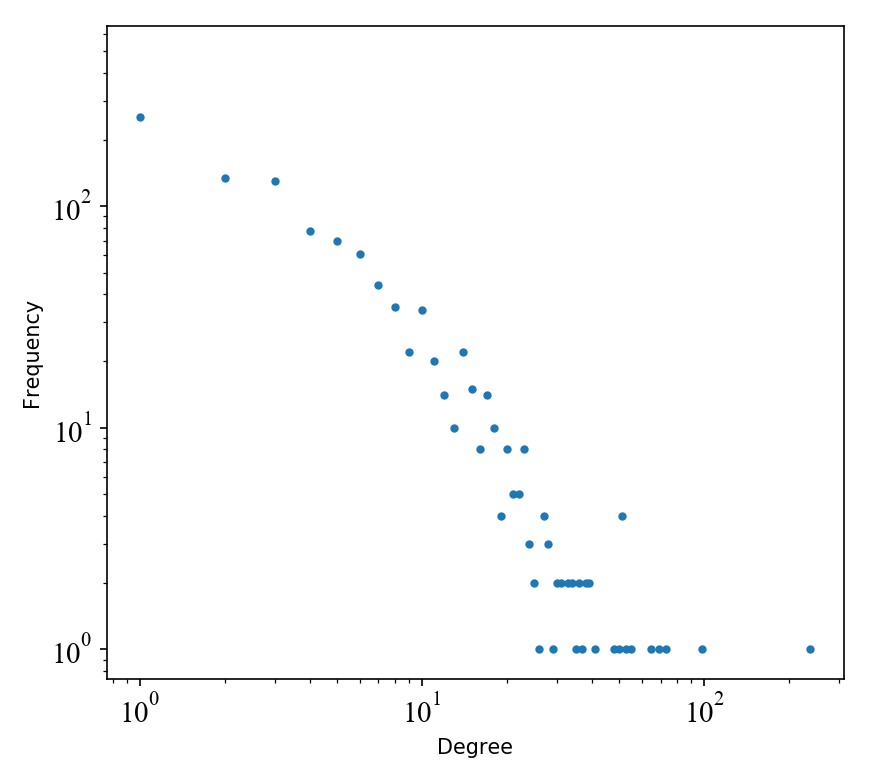出度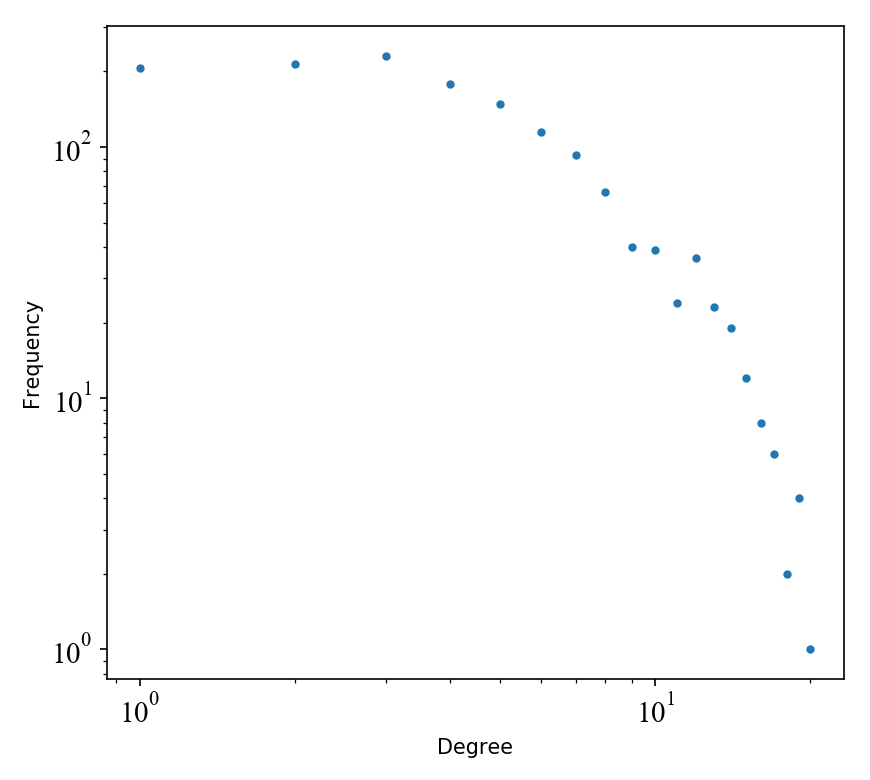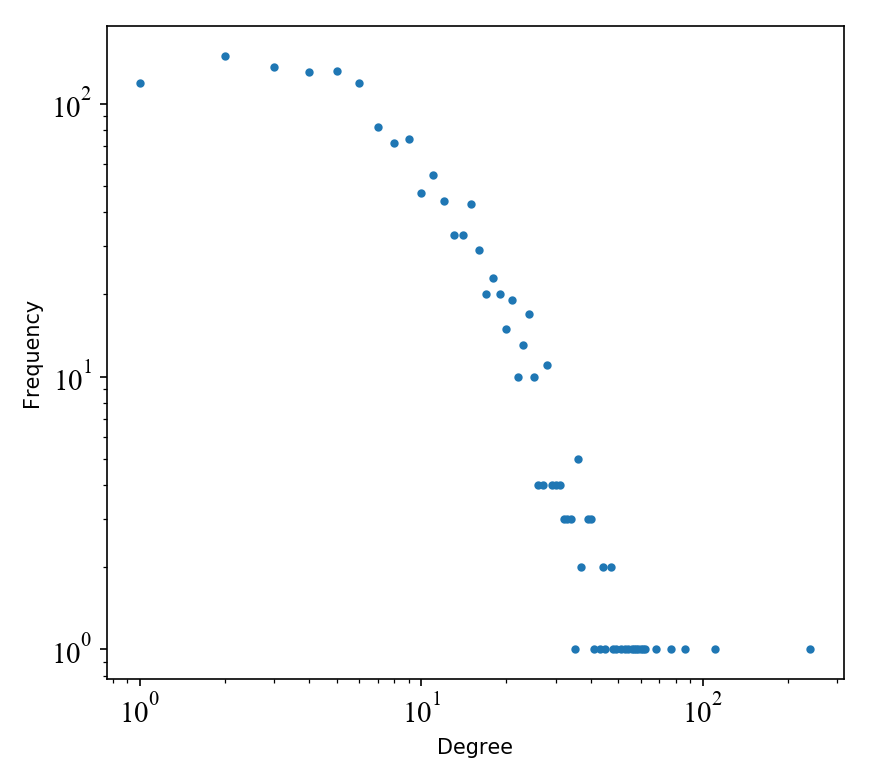展开全文可视化 python
• 求网络图中各节点的的分布曲线， 聚类系数及整个网络的聚类系数，和复杂网络中两节点的距离以及平均路径长度
• matlab函数求-相关性，也就是同配网络当 r > 0 -正相关 assortativeness，当 r -负相关 disassortativeness。
• function [DeD,aver_DeD]=Degree_Distribution(A) | 错误: 此上下文中不允许函数定义。 需要导入数据吗？复杂网络度的分布
• 这个Matlab程序是一个对复杂网络分布进行计算的程序，用于复杂网络的分析。
• 复杂网络聚类系数的matlab编程代码,将复杂网络存储为矩阵,再对其matLab编程,可得到分布图复杂网络
• 对于无标度网络，我们发现平均两步度随着度分布异质性的增加而增加，这表明平均两步度可用于测量复杂网络度分布的异质性。 另外，在给定节点平均度的条件下，我们可以通过最大化平均两步度来设计对随机故障具有最佳...研究论文
• 复杂网络中心性1. 中心（Degree Centrality）2. 介数中心（Betweenness Centrality）3. 接近中心（Closeness Centrality） 1. 中心（Degree Centrality） 中心（Degree Centrality）是在网络分析中...

1. 度中心性（Degree Centrality）

度中心性（Degree Centrality）是在网络分析中刻画节点中心性（Centrality）的最直接度量指标。一个节点的节点度越大就意味着该节点的度中心性越高，该节点在网络中就越重要。

某个节点 度中心性 计算公式如下：
D C i = k i N − 1 DC_i=\frac{k_i}{N-1}
其中：

• k i k_i 表示现有的与节点 i i 相连的边的数量
• N − 1 N-1 表示节点 i i 与其他节点都相连的边的数量2. 介数中心性（Betweenness Centrality）

节点介数是指一个网络里通过节点的最短路径条数

某个节点的 介数中心性 的计算公式如下：
B C i = ∑ s ≠ i ≠ t n s t i g s t BC_i=\sum_{s\neq i\neq t}\frac{n^i_{st}}{g_{st}}
其中：

• n s t i n^i_{st} 表示经过节点 i i ，且为最短路径的路径数量
• g s t g_{st} 表示连接 s s t t 的最短路径的数量

归一化（令结果 < 1）后，有：
B C i = 1 ( N − 1 ) ( N − 2 ) / 2 ∑ s ≠ i ≠ t n s t i g s t BC_i=\frac{1}{(N-1)(N-2)/2}\sum_{s\neq i\neq t}\frac{n^i_{st}}{g_{st}}上图计算节点 1 1 的介数中心性：

• 5 5 -> 4 4 ，最短路径为 ( 5 , 1 , 4 ) (5,1,4) ， 该路径经过节点 1 1 ，所以 n 54 1 = 1 , g 54 = 1 n^1_{54}=1,g_{54}=1
• 5 5 -> 3 3 ，最短路径为 ( 5 , 3 ) (5,3) ， 该路径不经过节点 1 1 ，所以 n 53 1 = 0 , g 53 = 1 n^1_{53}=0,g_{53}=1
• 5 5 -> 2 2 ，最短路径为 ( 5 , 1 , 2 ) , ( 5 , 3 , 2 ) (5,1,2),(5,3,2) ， 经过节点 1 1 的路径为 ( 5 , 1 , 2 ) (5,1,2) ，所以 n 52 1 = 1 , g 52 = 2 n^1_{52}=1,g_{52}=2
• 4 4 -> 3 3 ，最短路径为 ( 4 , 1 , 2 , 3 ) , ( 4 , 1 , 5 , 3 ) (4,1,2,3),(4,1,5,3) ， 两条路径都经过节点 1 1 ，所以 n 43 1 = 2 , g 43 = 2 n^1_{43}=2,g_{43}=2
• 4 4 -> 2 2 ，最短路径为 ( 4 , 1 , 2 ) (4,1,2) ， 该路径经过节点 1 1 ，所以 n 42 1 = 1 , g 42 = 1 n^1_{42}=1,g_{42}=1
• 3 3 -> 2 2 ，最短路径为 ( 3 , 2 ) (3,2) ， 该路径不经过节点 1 1 ，所以 n 32 1 = 0 , g 32 = 1 n^1_{32}=0,g_{32}=1
• 最后得出 B ( 1 ) = 7 2 B(1)=\frac{7}{2} ，对其归一化得 B ( 1 ) = 7 12 B(1)=\frac{7}{12}

3. 接近中心性（Closeness Centrality）

接近中心性用于衡量节点重要性

某个节点的 接近中心性 C C i CC_i 为：
d i = 1 N − 1 ∑ j = 1 N d i j C C i = 1 d i d_i=\frac{1}{N-1}\sum^{N}_{j=1}d_{ij} \quad \quad CC_i=\frac{1}{d_i}
其中 d i d_i 表示节点 i i 到其余各点的平均距离，平均距离的倒数就是接近中心度

例：以上图节点 A A 为例，图中点的个数 N = 11 N=11

• A A 相连的路径为 1 1 的共 4 4 个点，为 D , E , F , B D,E,F,B
• A A 相连的路径为 2 2 的共 3 3 个点，为 G . C , H G.C,H
• A A 相连的路径为 3 3 的共 3 3 个点，为 I , J , K I,J,K
• 可得 A A 的平均距离为 d ( A ) = 1 10 ( 4 + 2 ∗ 3 + 3 ∗ 3 ) d(A)=\frac{1}{10}(4+2 *3+3*3) A A 的接近中心度为 C C ( A ) = 1 d ( A ) CC(A)=\frac{1}{d(A)}
展开全文• 利用python载入邻接矩阵绘制网络图，基于python语言的特点，对邻接矩阵加以处理后再进行应用，即将邻接矩阵去除第一...在图论框架下, 可用不同的全局参量来表示复杂网络的特征, 最基本特征包括分布 P(k)和聚类系数C。
• 求网络图中各节点的的分布曲线， 聚类系数及整个网络的聚类系数，和复杂网络中两节点的距离以及平均路径长度
• 常见复杂网络分析方法

万次阅读 多人点赞 2019-09-18 15:38:07
常见的复杂网络分析方法基本分析方法关联分析方法 注：本文部分内容来自《复杂网络分析与应用》与《中国航空复杂网络的结构特征与应用分析》 1.赵正旭,郭阳,等.复杂网络分析与应用[M]北京:科学出版社,2018. 2.陈...

常见的复杂网络分析方法

注：本文部分内容来自《复杂网络分析与应用》与《中国航空复杂网络的结构特征与应用分析》
1.赵正旭,郭阳,等.复杂网络分析与应用[M]北京:科学出版社,2018.
2.陈航宇,李慧嘉.中国航空复杂网络的结构特征与应用分析[J].计算机科学,2019,s1:300-304.

1.基本分析方法

1.1.度和度的分布：
度是指与该节点直接连接的节点个数。度值的大小反映了节点在整个网络中的重要性。度分布p(k)反映了网络节点中度值为k的节点所占的比例。

1.2.平均路径长度
相互连接的两个节点i和j之间边数最少的路径所包含的边数即为这两个节点间的距离dij。取尽网络中任意两节点的组合，网络的平均路径长度定义为所有组合之距离的平均值：1.3.聚类系数
聚类系数Ci是指所有与节点i相连的节点之间实际相连的边数占这些点可能的最大连边数目的比例，聚类系数反映网络节点的聚类情况：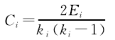其中，ki为与节点i连接的其他节点的个数；Ei为这ki个节点间相互连接的边数（两点之间的双向边和单向边都按1条计数）。整个网络C定义为网络中所有节点聚类系数的平均值：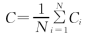其中，C取1说明网络中的所有节点都相连。

1.4.介数
网络中包含节点m的最短路径的条数定义为节点m的介数。介数的大小反映了节点在整个航空网络中的必要性和影响力，介数越大的节点在网络中的中枢性越强。（注意：介数与度值的概念，一个是必要性大，一个是重要性大）

1.5.核数
一个图的k-核是指反复去掉度小于或等于k的节点所剩余的子图。存在于k-核的节点，在（k+1）-核中被除去，则k就是该节点的核数。节点核数中的最大值称为网络的核数。节点的核数可以表明节点在核中的深度。图的核数大，说明图中大部分节点不会因为其他节点收到破坏而轻易脱离出网络，整个网络具有较深的层次。

2.关联分析方法

2.1.度度相关性
首先计算节点的邻点平均度：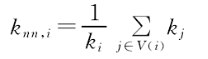其中，k为节点度值，节点j与节点i相连。将网络中节点度值都为k的所有节点Nk的邻节点的平均度knn,i取平均，得到度为k的节点的邻点平均度：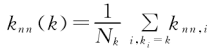如果knn(k)随k的增加而增加，即knn(k)-k曲线斜率大于零，则表示度大的节点倾向于连接其他度大的节点，成为度正相关（或同配性）；反之，如果knn(k)随k的增加而递减，表示度大的节点倾向于连接其他度小的节点，成为度负相关（或异配性）；如果knn(k)不随k的变化而变化，则称节点的度是不相关。

2.2.度权相关性
定义含权的节点i的所有邻节点j的平均加权度为：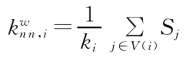分析邻节点平均加权度随节点的加权度值S的变化，以及邻节点平均加权度随节点的度值k的变化。
分别分析邻节点平均加权度与节点度值之间，邻节点平均加权度与节点的加权度值之间的关系。若均呈现负相关性，则说明度大的节点偏好连接加权度小的节点；且若邻节点平均加权度随着节点度值与节点加权度值的增大，相关性趋势减弱，则说明权值对节点间的影响较大。

2.3.介数相关性
介数相关性描述的是节点根据介数相互选择的偏好。一个介数为g的节点i，与j节点相连，则节点i的邻点平均介数记为：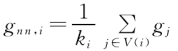将网络中节点介数都为g的所有节点Ng的邻节点的平均介数gnn，i进行平均，得到“介数为g的节点的邻点平均介数”：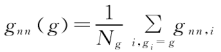分析网络节点的邻点平均介数gnn与介数g的分布关系，若呈现明显的负相关性，说明介数大的节点偏好连接其他介数小的节点，反之，则说明介数大的节点偏好连接其他介数大的节点。

2.4.簇度相关性
节点的邻节点相互连接的集聚程度与节点度值的相关性成为簇度相关性。分析节点的度与平均聚类系数之间的关系。若度值大，聚类系数小，则说明度值大的为网络的中心。

展开全文复杂网络
• 基于复杂网络节点集中和分散的无参数社区检测方法研究论文
• 计算复杂网络的相关特性，比如，，平均距离，最短路
• 一个节点的节点越大就意味着这个节点的中心性越高，该节点在网络中就越重要。 1.2 计算方法 在无向图（Undirected Graph）中，中心性测量网络中一个节点与所有其它节点相联系的程度。对于一个拥有g个节点的无...算法 python 机器学习 节点中心度
• 以自然连通为抗毁性谱测度指标,详细分析了分布、小世界性、关联性3种典型复杂网络结构属性对复杂网络抗毁性的影响:通过混合择优模型构造不同分布复杂网络,研究了分布对抗毁性的影响,研究表明在相同条件下,...
• 复杂网络简单指标：度度相关性、聚集系数的计算。改变网络结构：去掉网络的边或者节点的程序。
• 复杂网络介绍（一）

万次阅读 多人点赞 2018-10-11 15:21:05
复杂网络 1.定义： 复杂网络即呈现高度复杂性的网络，是复杂系统的抽象。 具有自组织、自相似、吸引子、小世界、无标度中部分或全部性质的网络称为复杂网络。 2．名词解释： 1）节点：由于复杂网络是复杂系统...
• 无论是实际网络还是对模型网络进行分析，都离不开对网络拓扑统计指标的计算。反映网络结构与动力学特性的统计指标有很多，Costa等的Characterization of Complex Networks: A Survey of measurements一文对此有全面...
• 原文地址：陈关荣老师整理的复杂网络的资源作者：zhengw789 http://www.ee.cityu.edu.hk/~gchen/ComplexNetworks.htm http://mrvar.fdv.uni-lj.si/sola/info4/programe.htm 原文地址：NetworkX的...
• Python复杂网络分析库networkx 看这一篇就够了

千次阅读 多人点赞 2020-01-26 01:47:30
networkx在2002年5月产生，是一个用Python语言开发的图论与复杂网络建模工具，内置了常用的图与复杂网络分析算法，可以方便的进行复杂网络数据分析、仿真建模等工作。 networkx支持创建简单无向图、有向图和多重图...算法 python 机器学习 大数据
• 各种复杂网络的matlab程序，包括求最大特征根，分布，聚类系数，点和边的介数，网络平均距离，ER,WS,BA网络生成等等。
• 对复杂网络的度分布进行详细研究，着重于度分布指数与网络拓扑结构的理论分析，并有实例验证，是复杂网络度分布研究中难得的经典文献，很有价值，值得学习...复杂网络 度分布
• 复杂网络度和度分布、最短路径、点介数、聚类系数代码
• 本科研论文研究了基类经典复杂网络的节点乘积分布，理论分析了节点乘积分布的表达式，并通过仿真实验进行了验证，适合复杂网络方向的研究者借鉴和参考复杂网络论文
• 关于复杂网络的节点重要的评估，主要是几个重要的评估方法复杂网络...